Chemical Equations: Writing & Balancing

# Chemical Equations: Writing & Balancing - Science Class 10

Balancing chemical equations involves the addition of stoichiometric coefficients to the reactants and products. This is important because a chemical equation must obey the law of conservation of mass and the law of constant proportions, i.e. the same number of atoms of each element must exist on the reactant side and the product side of the equation.

## Chemical Equation

• A chemical equation is a symbolic representation of a chemical reaction in which the reactants and products are denoted by their respective chemical formulae.
• An example of a chemical equation is 2H2 + O2 → 2H2O which describes the reaction between hydrogen and oxygen to form water
• The reactant side is the part of the chemical equation to the left of the ‘→’ symbol whereas the product side is the part to the right of the arrow symbol.
Reactant: The substance or substances which takes part in a chemical reaction are called reactants.
Products: The new substance or substances formed as a result of a chemical reaction are called products.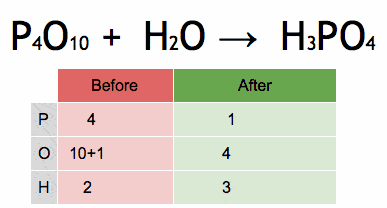In the above reaction, P4O10 & water are reactants and Phosphoric acid is the product.

Question for Chemical Equations: Writing & Balancing
Try yourself:
Why is it important to balance chemical equations?

## Rules for writing a word equation:

(a) The substances taking part in chemical reaction reactants are always written on the left-hand side of arrow.

(b) The substances formed after the chemical reaction, products are always written on the right hand side of arrow.

(c) A plus sign (+) is put in between the reactants or the products. If their number is two or more.

(d) An arrow (→) is put between the reactants and products, the arrow shows the direction of the reaction in which the reaction proceeds. The arrow is read as "to yield" or "to form".

In the word equation when symbols and chemical formulas of the reactants and products are used then it is called a chemical equation.

Example: Na + H2O → NaOH + H2
i.e. A chemical equation is a statement that describes a chemical reaction in terms of symbols and formulas. In this equation the law of conservation of mass is not obeying. Such chemical equation is called skeletal equation or unbalanced equation.
A chemical equation expressed in symbols and formula, such that the number of atoms of different elements towards the side of the reactants is not equal to the number of atoms of the products is called skeletal equation or unbalanced equation.
To make this equation meaningful, this equation is balanced then it is called balanced chemical equation.

### Charge Table: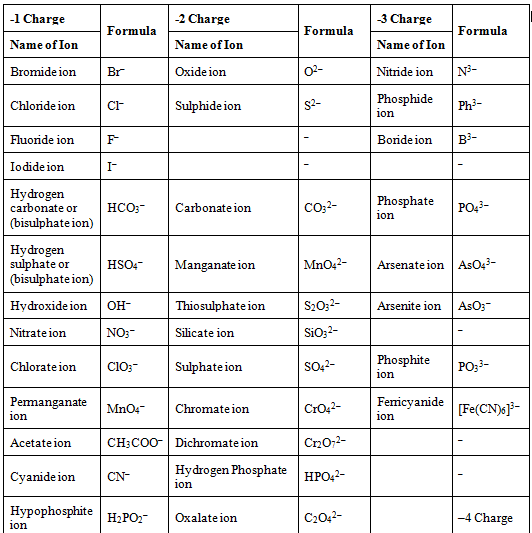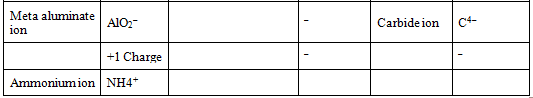## Balancing Chemical Equations

A balanced chemical equation is one that contains an equal number of atoms of each element on both sides of the equation.

The simple equations are balanced by the "hit and trial method" which is done in the following steps.

Step (i) Count the no. of atoms of various elements on both sides of the equation.

Example: Fe + H2O → Fe3O4 + H2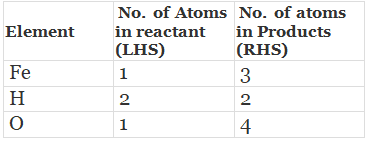Step (ii) Start balancing with the compound which contains maximum number of atoms. It may be a reactant or product. In that compound select the element which has maximum number of atoms.
According to this rule Fe3O4 has maximum number of atoms & oxygen has 4 atoms so it is selected.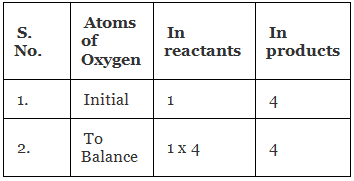So the partly balanced equation is
Fe + 4H2O → Fe3O4 + H2

Step (iii) Fe and H are not balanced in the above reaction so the above reaction repeat the above process for both i.e.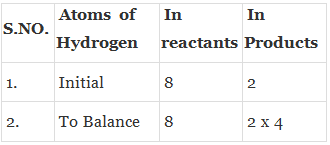Now the equation becomes as
Fe + 4H2O → Fe3O4 + 4H2

Step (iv) Balance the Iron atoms similarly.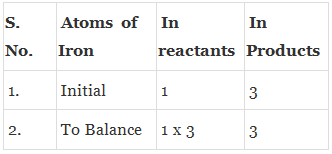Now the equation is
3Fe + 4H2O → Fe3O4 + 4H2

Step (v) Finally check the correctness of the balanced equation by counting the number of atoms on both sides of the equation.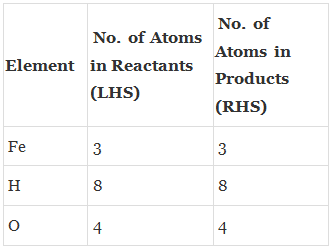Step (vi) To make chemical equation more informative physical states of the reactants and products are mentioned as for solid (s), liquid (l), gas (g) and for aqueous solution of reactant or product (aq) is written.
Now the equation becomes as
3Fe(s) + 4H2O(g) → Fe3O4(s) + 4H2(g)

Symbol (g) with water is written to show that water is used in the form of steam in this equation.
If gas is evolved in a reaction it can be shown by the symbol (↑) after the formula i.e. arrow pointing upwards e.g.
2Na(s) + 2H2O(l) → 2 NaOH(aq) + H2(g) or H2 (↑)
The symbol (↓) or ppt is be written for precipitate.
NaCl + AgNO3 → NaNO3 + AgCl (↓) or ppt.
A reversible reaction is represented by the (⇌) symbol and irreversible reaction by the (→) symbol.
The heat evolved in a chemical reaction is written on the right side by putting positive (+) sign and heat absorbed in the chemical reaction is written on the right hand side by putting negative (-) sign.
N2 + 3H2 ⇌ 2NH3 + 22400 calories (Exothermic reaction)
N2 + O2 ⇌ 2NO - 43200 calories (Endothermic reaction)

Sometimes the reaction conditions, such as temperature, pressure, catalyst etc. are written above or below the arrow in the equation e.g.
N2 + 3H2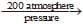2NH3 + 22400 calorie heat
450°C (Fe-Mo)

Exothermic and Endothermic Reactions: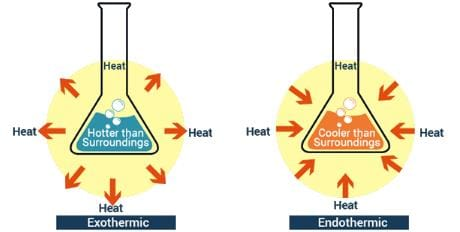Fig. Difference between endothermic and exothermic reactions

(i) Exothermic reaction: The reaction in which heat is liberated (or given out) is called an exothermic reaction.
Example: C(s) + O2(g) → CO2(g) + Heat (393 kJ/mol)
(ii) Endothermic reaction: The reaction in which heat is absorbed (or taken in ) is called an endothermic reaction.
Example: C(s) + 2S(g) → CS2(g) - Heat (92 kJ/mol)

The reaction with + Heat term on the product side is called exothermic reaction, while those with - Heat term on the product side are called endothermic reactions.
During respiration, the digested food gets oxidised and the energy is released. That is why, it is considered as an exothermic reaction.
Balancing of a chemical equation is necessary because no matter (hence, no atom) is lost or gained during a chemical reaction.

Question for Chemical Equations: Writing & Balancing
Try yourself:
How can a chemical equation be balanced?

### Writing the symbols of Physical States of substances in Chemical equation:

By writing the physical states of substances a chemical equation becomes more informative.

• Gaseous state is represented by symbol ‘g’
• Liquid state is represented by symbol ‘l’
• Solid state is written by symbol ‘s’
• Aqueous solution is written by symbol ‘aq’

Writing the condition in which reaction takes place: The condition is generally written above and/or below the arrow of a chemical equation.

Thus, by writing the symbols of physical state of substances and condition under which reaction takes place, a chemical equation can be made more informative.

Q.1. Translate the following statements into chemical equations and then balance them.
(a) Hydrogen gas combines with nitrogen to form ammonia
(b) Hydrogen sulphide gas burns in air to give water and sulphur dioxide.
(c) Barium chloride reacts with aluminium sulphate to give aluminium chloride and a precipitate of barium sulphate.
(d) Potassium metal reacts with water to give potassium hydroxide and hydrogen gas.
Ans.
(a) N2(g) + 3H2(g)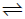2NH3(g)
(b) 2H2S + 3O2 → 2H2O + 2SO2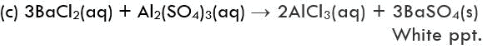(d) 2K (s) + 2H2O (1) → 2KOH + H2 (g)

Q.2. Balance the following chemical equations.
(a) HNO3 + Ca(OH)2 → Ca(NO3)2 + H2O
(b) NaOH + H2SO4 → Na2SO4 + H2O
Ans.
(a) 2HNO3 + Ca(OH)2 → Ca(NO3)2 + 2H2O
(b) 2NaOH + H2SO4 → Na2SO4 + 2H2O

Q.3. Write the balanced chemical equations for the following reactions.
(a) Calcium hydroxide + Carbon dioxide → Calcium carbonate + water

(b) Zinc + Silver nitrate → Zinc nitrate + Silver
(c) Aluminium + copper chloride → Aluminium chloride + Copper
(d) Barium chloride + Potassium sulphate → Barium sulphate + Potassium chloride.
Ans.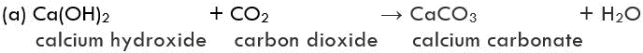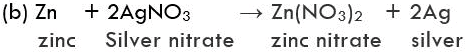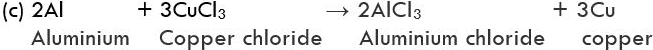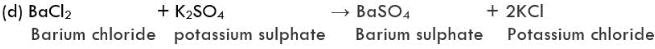Q.4. Write the balanced chemical equation for the following and identify the type of reaction in each case -
(a) Potassium bromide(aq) + Barium iodide(aq) → Potassium iodide(aq) + Barium bromide(s)
(b) Zinc carbonate (s) → Zinc oxide (s) + Carbon dioxide(g)
(c) Hydrogen(g) + Chlorine(g) → Hydrogen chloride(g)
(d) Magnesium(s) + Hydrochloric acid(aq) → Magnesium chloride(aq) + Hydrogen(g)
Ans.
(a) 2KBr(aq) + BaI2(aq) → 2KI(aq) + BaBr2(aq)
This reaction is a double-displacement reaction.
(b) ZnCO3(s) → ZnO(s) + CO2(g)
This reaction is a decomposition reaction

Q.5. Why should a magnesium ribbon be cleaned before burning in air?
Ans.
Magnesium reacts with the constituent gases of the atmosphere to form various compounds which get deposited over its surface. The ribbon is cleaned before burning to remove the layer of these compounds so that pure magnesium can burn in air.

The document Chemical Equations: Writing & Balancing | Science Class 10 is a part of the Class 10 Course Science Class 10.
All you need of Class 10 at this link: Class 10

## Science Class 10

78 videos|509 docs|153 tests

## FAQs on Chemical Equations: Writing & Balancing - Science Class 10

 1. What is a chemical equation?Ans. A chemical equation is a symbolic representation of a chemical reaction, which shows the reactants and products involved in the reaction, along with their respective chemical formulas and the relative amounts in which they combine.
 2. What are the rules for writing a word equation?Ans. The rules for writing a word equation are as follows: (1) Write the reactants on the left-hand side and the products on the right-hand side of the equation. (2) Use appropriate chemical symbols and formulas for the reactants and products. (3) Use "+" to separate two or more reactants or products. (4) Use "->" to represent the direction of the reaction. (5) Use state symbols (s, l, g, aq) to indicate the physical state of each reactant and product.
 3. Why is balancing a chemical equation important?Ans. Balancing a chemical equation is important because it ensures that the law of conservation of mass is obeyed. This law states that matter cannot be created or destroyed in a chemical reaction, only rearranged. By balancing the equation, we make sure that the same number of each type of atom is present in the reactants and products, which means that no atoms are lost or gained during the reaction.
 4. What is the process for balancing a chemical equation?Ans. The process for balancing a chemical equation involves the following steps: (1) Write the unbalanced equation. (2) Count the number of atoms of each element present in the reactants and products. (3) Add coefficients (whole numbers) to the reactants and/or products to balance the number of atoms of each element. (4) Check that the number of atoms of each element is the same on both sides of the equation. (5) If necessary, repeat steps 3 and 4 until the equation is balanced.
 5. What are some common mistakes to avoid when balancing a chemical equation?Ans. Some common mistakes to avoid when balancing a chemical equation are: (1) Forgetting to check that the equation is balanced after adding coefficients. (2) Using fractions or decimals for coefficients instead of whole numbers. (3) Changing the subscripts of the chemical formulas to balance the equation. (4) Balancing only one element at a time instead of considering all the elements present. (5) Forgetting to include state symbols, which can affect the coefficients needed for balancing.

## Science Class 10

78 videos|509 docs|153 testsExplore Courses for Class 10 examSignup to see your scores go up within 7 days! Learn & Practice with 1000+ FREE Notes, Videos & Tests.
10M+ students study on EduRev
Track your progress, build streaks, highlight & save important lessons and more!
Related Searches

,

,

,

,

,

,

,

,

,

,

,

,

,

,

,

,

,

,

,

,

,

;